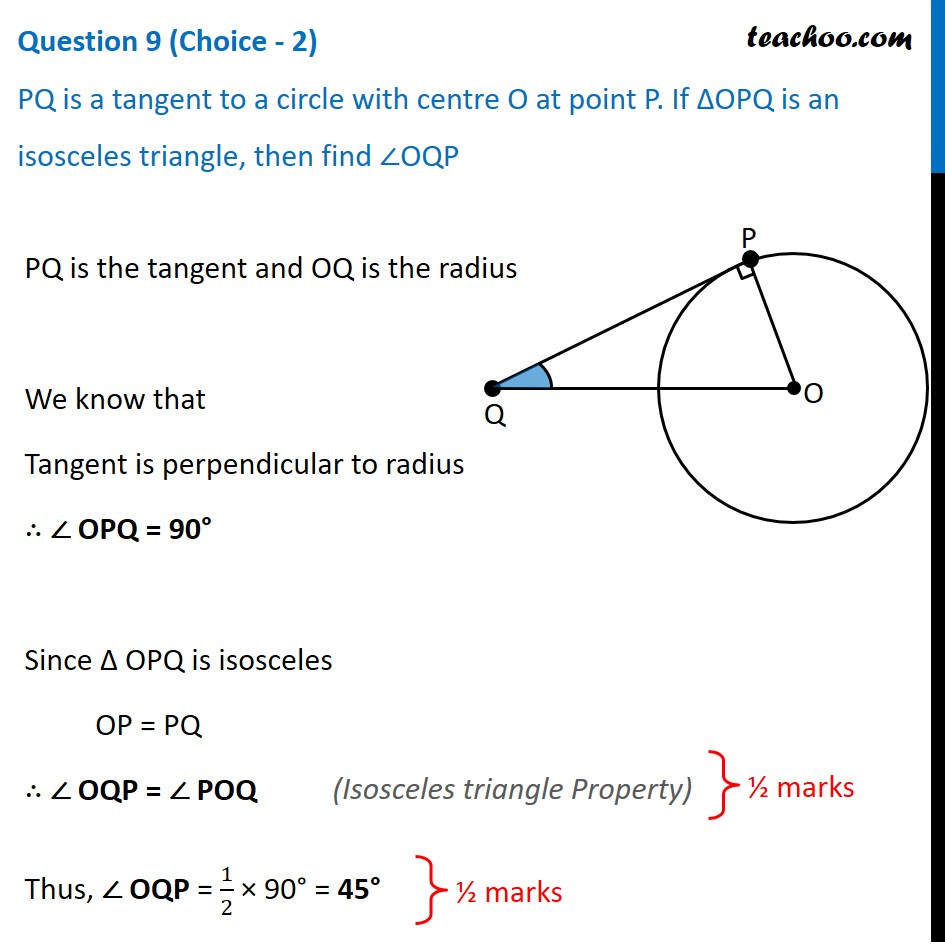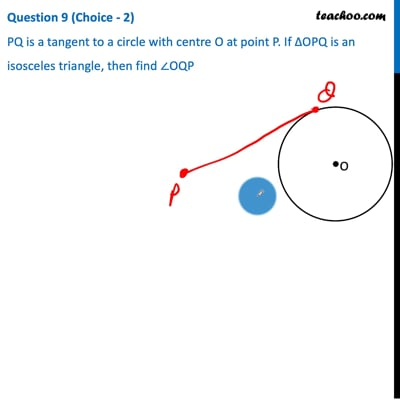CBSE Class 10 Sample Paper for 2021 Boards - Maths Standard

Class 10
Solutions of Sample Papers for Class 10 Boards

## PQ is a tangent to a circle with centre O at point P. If ∆OPQ is an isosceles triangle, then find ∠OQPThis video is only available for Teachoo black users

Introducing your new favourite teacher - Teachoo Black, at only ₹83 per month

### Transcript

Question 9 (Choice - 2) PQ is a tangent to a circle with centre O at point P. If ∆OPQ is an isosceles triangle, then find ∠OQPPQ is the tangent and OQ is the radius We know that Tangent is perpendicular to radius ∴ ∠ OPQ = 90° Since Δ OPQ is isosceles OP = PQ ∴ ∠ OQP = ∠ POQ Thus, ∠ OQP = 1/2 × 90° = 45° (Isosceles triangle Property)